#### 期刊菜单

Modeling and Optimization of a 36 kVA High-Frequency Transformer
DOI: 10.12677/MOS.2021.101008, PDF, HTML, XML, 下载: 364  浏览: 571

Abstract: In the fields of DC transmission, photovoltaic power generation and electric locomotive traction, the power electronic technology provides a critical support. Furthermore, the high-frequency trans-former is a key component of the power electronic equipment. In recent years, with the application of nanocrystalline materials in manufacture of high-frequency transformers, the performance of transformers has been greatly improved. However, there are still many difficulties in the design of high-frequency transformers with relatively large power in terms of reducing parasitic parameters and increasing power density. This research uses the nanocrystalline as the magnetic core, modeling a 36 kVA transformer with10 kHz and making numerical optimization of total loss and parasitic parameters. Finally, computer simulation technology was used to verify the feasibility and validity of the optimization, and the optimal design parameters were obtained by the comparison.

1. 引言

2. 高频变压器的建模Table 1. High frequency transformer parameters

2.1. 高频变压器的数学模型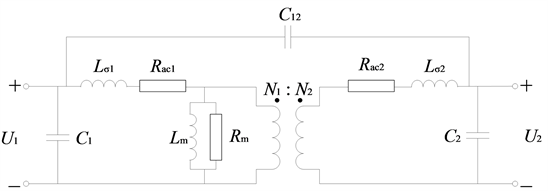Figure 1. General equivalent circuits of the high frequency transformer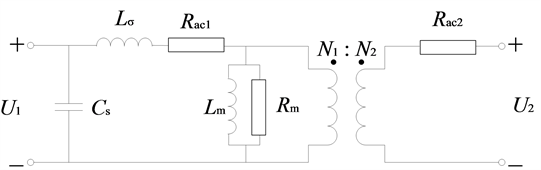Figure 2. Simplified equivalent circuit of the High-frequency transformer

${L}_{\sigma }={L}_{\sigma 1}+\frac{{N}_{1}^{2}}{{N}_{2}^{2}}{L}_{\sigma 2}$ (1)

${C}_{s}={C}_{1}+\frac{{N}_{2}^{2}}{{N}_{1}^{2}}{C}_{2}+{\left(\frac{{N}_{2}}{{N}_{1}}-1\right)}^{2}{C}_{12}$ (2)

2.2. 最大磁通密度Bm的选择

${P}_{c}={k}_{c}{f}^{\alpha }{B}_{m}^{\beta }{V}_{c}{k}_{f}$ (3)

${P}_{m}={R}_{ac1}{I}_{1}^{2}+{R}_{ac2}{I}_{2}^{2}={F}_{r1}{N}_{1}\frac{\rho {l}_{m1}}{{S}_{1}}{I}_{1}^{2}+{F}_{r2}{N}_{2}\frac{\rho {l}_{m2}}{{S}_{2}}{I}_{2}^{2}$

$\left( 5.35 \right)$

${N}_{1}=\frac{{U}_{1}}{4{B}_{m}{A}_{c}f},\text{\hspace{0.17em}}\text{\hspace{0.17em}}{N}_{2}=\frac{{U}_{2}}{4{B}_{m}{A}_{c}f}$ (4)

${P}_{s}={P}_{c}+{P}_{m}$ (5)

${B}_{z}^{\beta +1}=\frac{{F}_{r1}{U}_{1}\frac{\rho {l}_{m1}}{{S}_{1}}{I}_{1}^{2}+{F}_{r2}{U}_{2}\frac{\rho {l}_{m2}}{{S}_{2}}{I}_{2}^{2}}{4{A}_{c}{f}^{\alpha +1}{k}_{m}\beta {V}_{c}{k}_{f}}$ (6)

2.3. 建模与理论参数计算Figure 3. CC type with nanocrystal coresTable 2. Diameter of nanocrystalline CC coresTable 3. Parameters of nanocrystalline CC cores

${N}_{1}=\frac{{U}_{1}}{4{B}_{m}{A}_{c}f}=48\text{ }匝$ (7)

${N}_{2}=\frac{{U}_{2}}{4{B}_{m}{A}_{c}f}=16\text{ }匝$ (8)

${I}_{1}=\frac{P}{{U}_{1}}=40\text{\hspace{0.17em}}\text{A}$ (9)

${I}_{2}=\frac{P}{{U}_{2}}=120\text{\hspace{0.17em}}\text{A}$ (10)

$\delta =\frac{66.1}{\sqrt{f}}=\frac{66.1}{\sqrt{10000}}=0.661\text{\hspace{0.17em}}\text{mm}$ (11)

${S}_{1}=\frac{{I}_{1}}{J}=16\text{\hspace{0.17em}}{\text{mm}}^{2}$ (12)

${S}_{2}=\frac{{I}_{2}}{J}=48\text{\hspace{0.17em}}{\text{mm}}^{2}$ (13)Table 4. Winding type and winding parameters

${h}_{1}={h}_{2}=104×1.15=119.6\text{\hspace{0.17em}}\text{mm}<120\text{\hspace{0.17em}}\text{mm}$ (14)

$d=\left(2+1.23×3+0.92×2×2+2×4+3\right)×2=40.74\text{\hspace{0.17em}}\text{mm}<45\text{\hspace{0.17em}}\text{mm}$ (15)

${F}_{r1}=6.08,\text{\hspace{0.17em}}\text{\hspace{0.17em}}{F}_{r2}=1.87$ (16)

${R}_{dc1}=\frac{{l}_{1}}{\gamma {S}_{1}}=\frac{16×\left(196.43+216.73+237.02\right)}{5.8×{10}^{7}×16}×100=0.0112\text{\hspace{0.17em}}\Omega$ (17)

${R}_{dc2}=\frac{{l}_{2}}{\gamma {S}_{2}}=\frac{8×\left(259.23+283.36\right)}{5.8×{10}^{7}×48}×100=0.0037\text{\hspace{0.17em}}\Omega$ (18)

${R}_{ac1}={F}_{r1}{R}_{dc1}=0.0681\text{\hspace{0.17em}}\Omega$ (19)

${R}_{ac2}={F}_{r2}{R}_{dc2}=0.0070\text{\hspace{0.17em}}\Omega$ (20)

Table 5. Theoretical calculation parameters of high frequency transformer with equivalent circuitsTable 6. Performance of theoretical model of the high frequency transformer

3. 高频变压器的优化与仿真验证

3.1. 高频变压器优化方程

$x=\left(J;{d}_{j}\right)\in {ℝ}^{2}$ (21)

$\mathrm{min}{P}_{s}\left( x \right)$

$\text{s}\text{.t}\text{.}\text{\hspace{0.17em}}d\left(x\right)\le 45$

${d}_{1}\left(x\right)\le 2\delta$

${d}_{2}\left(x\right)\le 4\delta$

$\Delta \tau \left(x\right)\le 40$

$\eta \ge 0.99$

$\Delta U\left(x\right)\le 0.01$

${d}_{j}\ge 1.5$

$J\le 5$

$\mathrm{min}{L}_{\sigma }\left( x \right)$

$\text{s}\text{.t}\text{.}\text{\hspace{0.17em}}d\left(x\right)\le 45$

${d}_{1}\left(x\right)\le 2\delta$

${d}_{2}\left(x\right)\le 4\delta$

$\Delta \tau \left(x\right)\le 40$

$\eta \ge 0.99$

$\Delta U\left(x\right)\le 0.01$

${d}_{j}\ge 1.5$

$J\le 5$

3.2. 高频变压器优化结果Table 8. Performance of the optimized high frequency transformer

3.3. 仿真验证高频变压器优化结果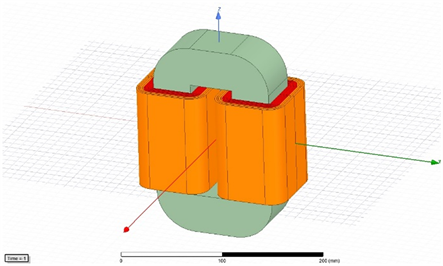Figure 4. High frequency transformer model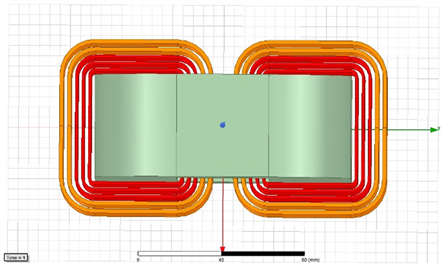Figure 5. High frequency transformer winding model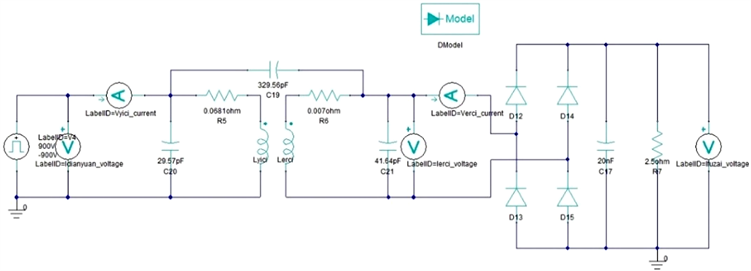Figure 6. Excitation external circuit of high-frequency transformer (rated state)

3.4. 初始高频变压器模型仿真结果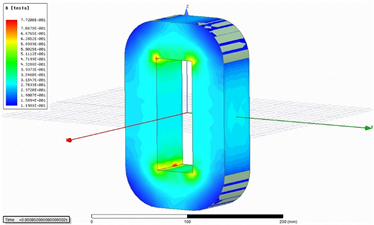Figure 7. Flux density distribution of the high frequency transformer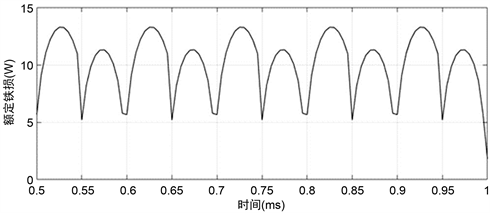Figure 8. Iron loss waveform of the high frequency transformerTable 9. Initial transformer simulation model circuit parameterTable 10. Performance of Initial Transformer simulation

3.5. 优化后高频变压器仿真结果Table 11. Optimized transformer simulation circuit parametersTable 12. Performance of the optimized transformer simulation

3.6. 检验漏感优化效果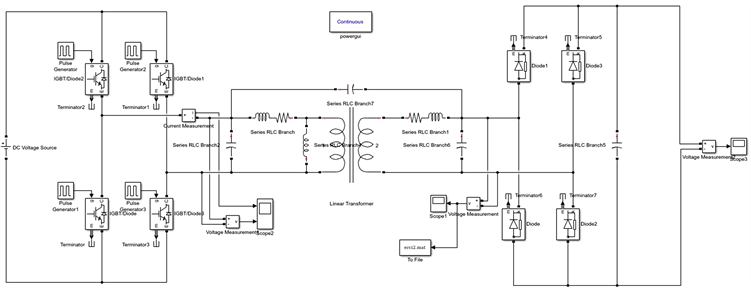Figure 9. High-frequency transformer no-load simulation circuitTable 13. Equivalent circuit distribution parameters for high frequency transformers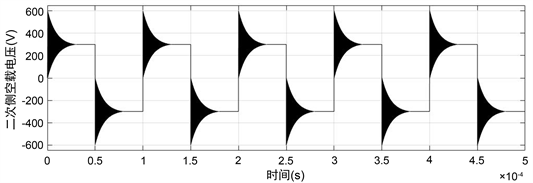Figure 10. Secondary voltage waveform of initial model with no-load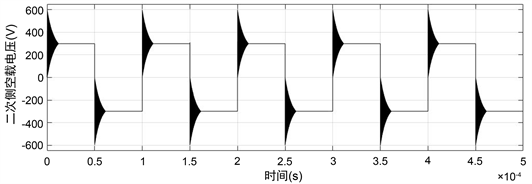Figure 11. Secondary voltage waveform of optimized model with no-load

4. 结论

  薛伟, 郑丽君, 高云广, 李婧, 张晓伟, 宋建成. 电力电子变压器中高频变压器的设计方法[J]. 电测与仪表, 2015, 52(23): 117-121.  Han, J.X., Kong, X.P., Li, P., Zhang, Z. and Yin, X.G. (2019) A Novel Low Voltage Ride through Strategy for Cascaded Power Electronic Transformer. Protection and Control of Modern Power Systems, 4, 1-12. https://doi.org/10.1186/s41601-019-0137-1  曹小鹏. 大功率高频变压器优化设计[D]: [硕士学位论文]. 南京: 东南大学, 2018.  叶栋. 高频变压器分布参数的确定及其影响分析[D]: [硕士学位论文]. 温州: 温州大学, 2018.  Balci, S., Sefa, I. and Altin, N. (2016) An Investigation of Ferrite and Nanocrystalline Core Materials for Medium-Frequency Power Transformers. Journal of Electronicmaterials, 45, 276-285.  张宁. 高频变压器优化设计方法与应用研究[D]: [硕士学位论文]. 北京: 华北电力大学, 2016.  张朋朋. 大功率高频开关电源变压器的优化设计[D]: [硕士学位论文]. 成都: 西南交通大学, 2009.